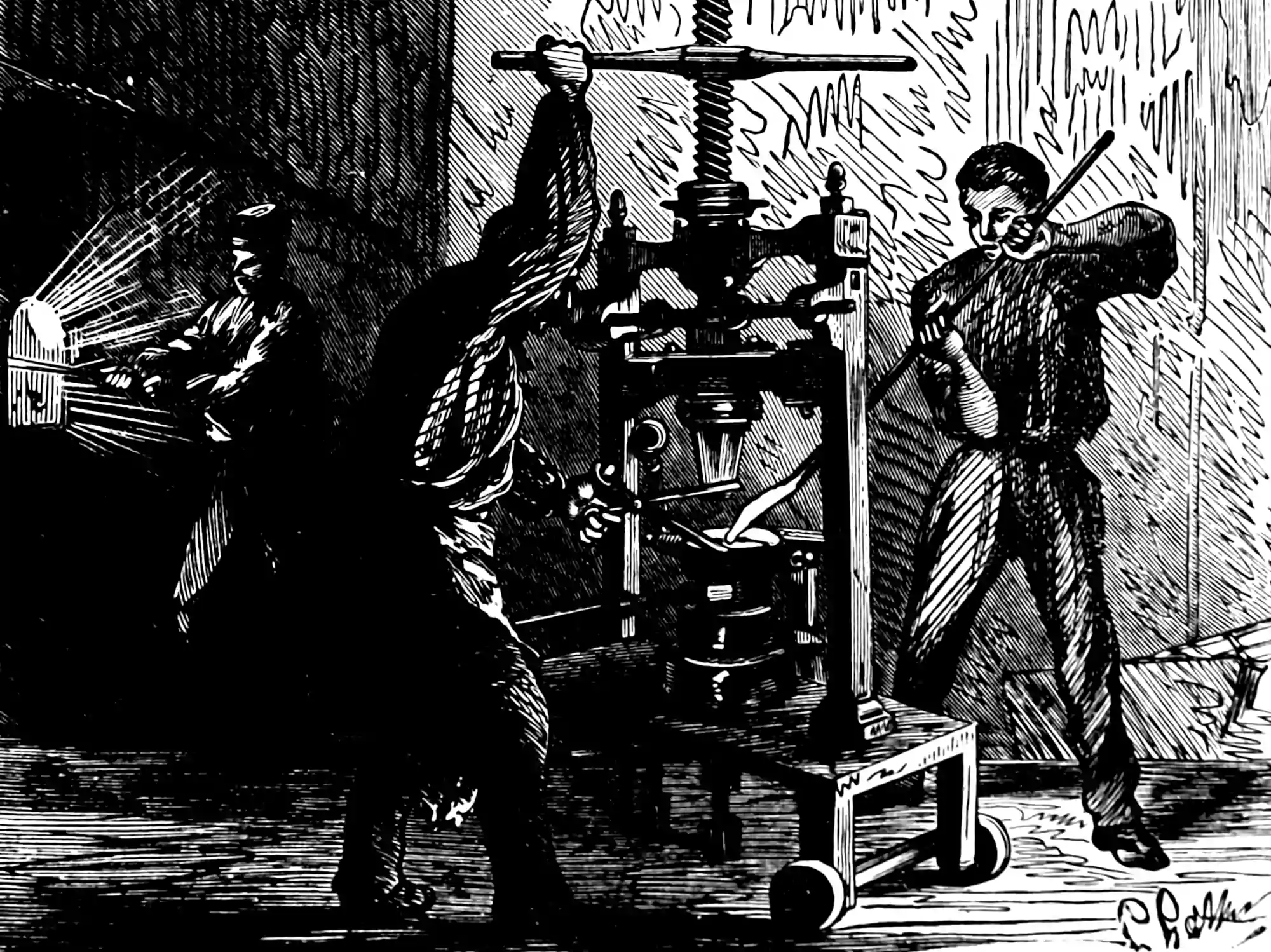# Conditional expectation and probability

Things I would like to re-derive for my own entertainment:

Conditioning in the sense of measure-theoretic probability. Kolmogorov formulation. Conditioning as Radon-Nikodym derivative. Clunkiness of definition due to niceties of Lebesgue integration.

TBC

## Nonparametric

Conditioning in full measure-theoretic glory for Bayesian nonparametrics. E.g. conditioning of Gaussian Processes is also fun.

## Disintegration

Chang and Pollard (1997), Kallenberg (2002).

## BLUE in Gaussian conditioning

e.g. Wilson et al. (2021):

Let $$(\Omega, \mathcal{F}, \mathbb{P})$$ be a probability space and denote by $$(\boldsymbol{a}, \boldsymbol{b})$$ a pair of square integrable, centered random variables on $$\mathbb{R}^{n_{a}} \times \mathbb{R}^{n_{b}}$$. The conditional expectation is the unique random variable that minimizes the optimization problem $\mathbb{E}(\boldsymbol{a} \mid \boldsymbol{b})=\underset{\hat{\boldsymbol{a}}=f(\boldsymbol{b})}{\arg \min } \mathbb{E}(\hat{\boldsymbol{a}}-\boldsymbol{a})^{2}$ In words then, $$\mathbb{E}(\boldsymbol{a} \mid \boldsymbol{b})$$ is the measurable function of $$\boldsymbol{b}$$ that best predicts $$\boldsymbol{a}$$ in the sense of minimizing the mean square error $$(6)$$.

Uncorrelated, jointly Gaussian random variables are independent. Consequently, when $$\boldsymbol{a}$$ and $$\boldsymbol{b}$$ are jointly Gaussian, the optimal predictor $$\mathbb{E}(\boldsymbol{a} \mid \boldsymbol{b})$$ manifests as the best unbiased linear estimator $$\hat{\boldsymbol{a}}=\mathbf{S} \boldsymbol{b}$$ of $$\boldsymbol{a}$$### No comments yet. Why not leave one?

GitHub-flavored Markdown & a sane subset of HTML is supported.